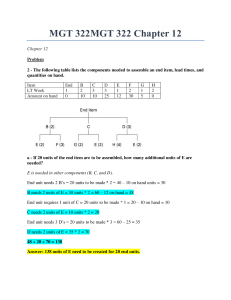# MGT 322 Chapter 12. Questions and Answers, with working.

PLDZ-48 In Stock
\$ 12.00 USD
Description

CLICK ON THE PRODUCT INFORMATION URL FOR MORE ON THE MGT 322

MGT 322MGT 322 Chapter 12 Chapter 12 Problem 2 - The following table lists the components needed to assemble an end item, lead times, and quantities on hand. Item End B C D E F G H LT Week 1 2 3 3 1 2 1 2 Amount on hand 0 10 10 25 12 30 5 0 a - If 20 units of the end item are to be assembled, how many additional units of E are needed? E is needed in other components (B, C, and D). End unit needs 2 B’s = 20 units to be made * 2 = 40 – 10 on hand units = 30 B needs 2 units of E = 30 units * 2 = 60 – 12 on-hand = 48 End unit requires 1 unit of C = 20 units to be made * 1 = 20 – 10 on hand = 10 C needs 2 units of E = 10 units * 2 = 20 End unit needs 3 D’s = 20 units to be made * 3 = 60 – 25 = 35 D needs 2 units of E = 35 * 2 = 70 48 + 20 + 70 = 138 b - An order for the end item is scheduled to be shipped at the start of week 11. What is the latest week that the order can be started and still be ready to ship on time? There are three sections that need to be finished in order for the end item to be complete i.e. (1) B, E, and F (2) C, G, and E, (3) D, H, and E. Lead times are as follows: (1) B = 2 weeks, E = 1 week, and F = 2 week, which is a total of 5 weeks (2) C = 3 weeks, G = 1 week, and E = 1 week, which is a total of 5 weeks (3) D = 3 weeks, H = 2 weeks, and E = 1 week, which is a total of 6 weeks Week 11 – 6 week lead time = 5 3 – The following table lists the components needed to assemble an end item, lead times (in weeks), and quantities on hand. a - If 40 units of the end item are to be assembled, how many additional units of B are needed?? End unit needs 2 L’s = 40 * 2 = 80 – 10 on hand = 70 L needs 2 units of B = 70 * 2 = 140 – 30 on hand = 110 End unit needs 1 C = 40 * 1 = 40 – 15 on hand = 25 C needs 2 units of B = 25 * 2 = 50 End unit needs three K’s = 40 * 3 = 120 – 20 on hand = 100 K needs 2 units of B = 100 * 2 = 200 110 + 50 + 200 = 360 b - An order for the end item is scheduled to be shipped at the start of week 8. What is the latest week that the order can be started and still be ready to ship on time? There are three sections that need to be finished in order for the end item to be complete i.e. (1) L, B, and J (2) C, G, and B, (3) K, H, and B. Lead times are as follows: (1) L = 2 weeks, B = 2 weeks, and J = 3 weeks, which is a total of 7 weeks (2) C = 3 weeks, G = 3 weeks, and B = 2 weeks, which is a total of 8 weeks (3) K = 3 weeks, H = 2 weeks, and B = 2 weeks, which is a total of 7 weeks Show Less

Recent Reviews Write a Review
0 0 0 0 reviews